Electron. J. Diff. Eqns., Vol. 2008(2008), No. 100, pp. 1-8.

### Multiplicity results for fourth-order boundary-value problem at resonance with variable coefficients Ling Xu

Abstract:
This paper studies the multiplicity of solutions for the fourth-order boundary value problem at resonance with variable coefficients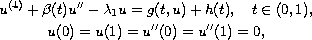where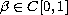with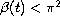on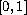,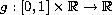is bounded continuous function,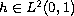and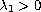is the first eigenvalue of the associated linear homogeneous boundary value problem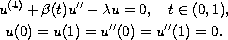The proof of our main result is based on the connectivity properties of the solution sets of parameterized families of compact vector fields.

Submitted April 14, 2008. Published July 30, 2008.
Math Subject Classifications: 39A10.
Key Words: Connected subsets; resonance; multiplicity results.

Show me the PDF file (220 KB), TEX file, and other files for this article.Ling Xu College of Mathematics and Information Science Northwest Normal University Lanzhou, Gansu 730070, China email: xuling_216@yahoo.cn